Technical Article

# Learn Stub Tuning With a Smith Chart

April 21, 2023 by Dr. Steve Arar

## Learn about impedance matching stub tuning using a Smith chart, as well as single and double stub tuning, impedance contour, and the "forbidden region" of a Smith chart.

In this article, you'll find an overview of stub tuning examples using the Smith chart to match RF transmission lines to various loads. Having the ability to design these matching circuits using a Smith chart can be handy if you ever find yourself working in the RF field because it is often intuitive and faster than calculating the analytical equations. The Smith chart was an invention of the electrical engineer Phillip Hagar Smith. In another article, we discuss how a shunt stub can be used to provide impedance matching. Additionally, impedance matching can also be achieved through a series stub, as we’ll discuss shortly. However, single stub matching cannot be used if the load impedance has some variations. In these cases, a better option is to use a double stub matching network, which is the focus of this article.

### Single Stub Tuning—Shunt and Series Configurations

In RF engineering, it is critical to match the line to its load. Impedance matching can be accomplished using a variety of different methods and components, each suited to specific applications. This, of course, leads to the inevitable and age-old dilemma where the engineer will have to balance the best possible solution with the cheapest possible implementation.

Stub tuning is one method that satisfies both of these criteria. In general, stub tuning is simply the process of adding a length of the transmission line to the existing length in either series or shunt circuit configuration to match the line to the load. This is achieved by placing a specific length of stub a specific distance away from the load. Figure 1 shows the two basic layouts of stub tuners, namely the shunt stub (Figure 1(a)) and series stub (Figure 1(b)) matching networks.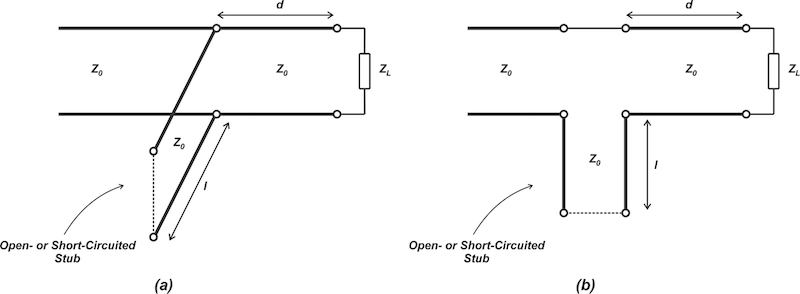##### Figure 1. Diagrams for a shunt stub (a) and series stub (b) matching networks.

The shunt stub method, which will be discussed in greater detail in another article, is overwhelmingly more popular. This is due to the fact that a shunt configuration doesn’t require breaking the existing connections and, thus, can be mechanically more convenient. For completeness, however, we’ll briefly discuss the series configuration through an example.

### Example 1: A Series Single Stub Matching Network

Design the series single stub matching network of Figure 1(b) to transform ZL = 100 + j50 Ω to ZIn = 50 Ω. Assume that the stub is terminated in a short. If the load impedance is a series RL circuit and the match frequency is 1 GHz, plot the reflection coefficient magnitude versus frequency.

The normalized load impedance z= 2 + j is marked on the following Smith chart in Figure 2.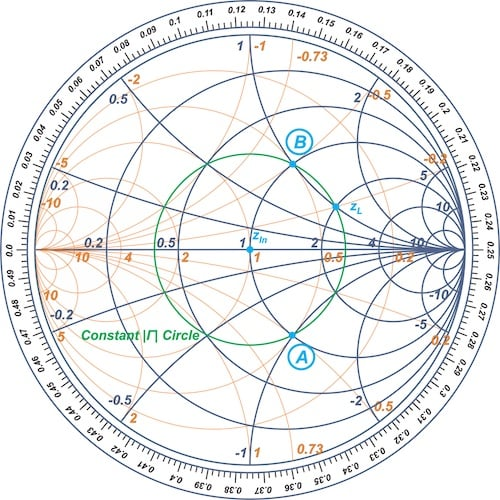##### Figure 2. A normalized load impedance zL = 2 + j on a Smith chart.

The matching circuit in Figure 1(b) starts with a series line of length, d, on the load side. We know that moving away from a termination on a transmission line produces a clockwise rotation along the load’s constant |Γ| circle. Therefore, the locus of the impedance seen at the input of the series line is the load’s constant |Γ| circle—the green circle in the above figure.

The addition of the stub only changes the reactive component of the impedance. Therefore, the length, d, should be chosen to move us to the constant-resistance circle of r = 1. Next, the stub reactance can cause us to traverse along the r = 1 circle to the center of the Smith chart. Here there are two intersection points—denoted as points A and B—in the above figure. Using point A, we obtain the path shown below shown in Figure 3.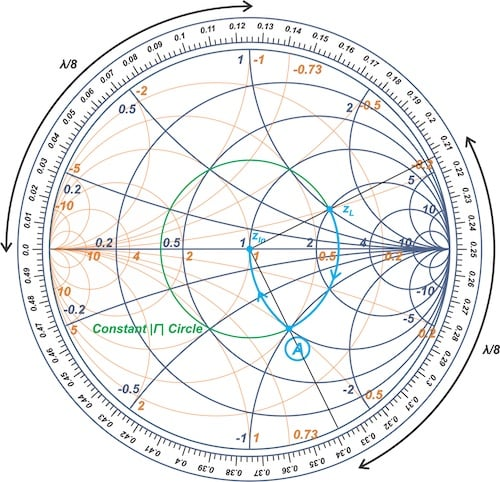##### Figure 3.The path on Smith chart for point A.

To find the length of the series line, d, we can draw lines from the center through zL and point A outward to the edge of the chart and measure the corresponding arc, leading to d = λ/8. To find the length of the stub, we should consider the impedance of point A. The normalized impedance at point A is 1 - j. Thus, the stub should provide a reactance of +j to move us to the center of the Smith chart. With a short-circuited stub, the length of the stub works out to λ/8, as illustrated above. The final matching circuit is shown in Figure 4.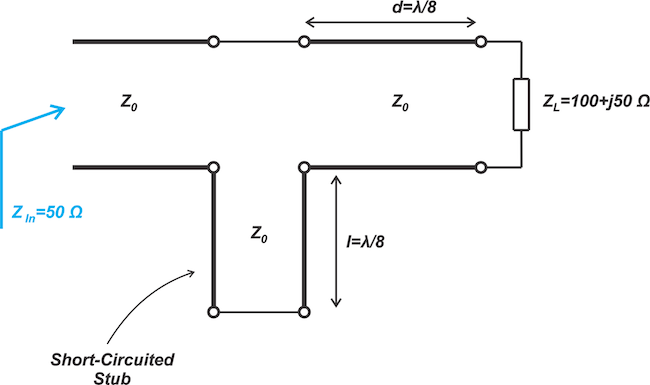##### Figure 4. Diagram for the final matching circuit for our first example.

Since Z= 100 + j50 Ω is said to be a series RL circuit and the match frequency is 1 GHz, we obtain R = 100 Ω and L = 7.96 nH. The input reflection coefficient (S11) of the circuit, shown below in Figure 5, confirms an excellent match at 1 GHz.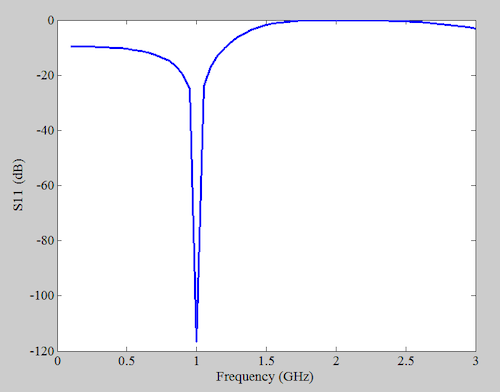##### Figure 5. Chart showing the input reflection coefficient vs frequency at 1 GHz.

Now let’s learn about the double stub tuning technique.

### Basic Idea Behind Double Stub Matching Networks

A single stub tuner can provide impedance matching between any values of input and load impedances as long as they have a non-zero real part. That’s why single stub tuning is quite effective and versatile; however, it has one drawback that can pose a problem in certain applications: both the stub position and length depend on the load impedance. As an example, consider the matching network shown below (Figure 6).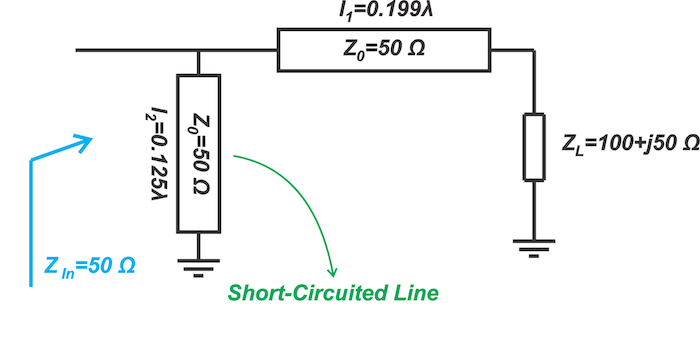##### Figure 6. Example matching network diagram.

The stub is at a distance of l1 = 0.199λ from the load. If the load impedance is not constant or changes because of manufacturing tolerances, then we have to adjust both l1 and l2. This is also true for the other type of shunt stub tuner that places the stub at the load end of the line. An example is shown in Figure 7.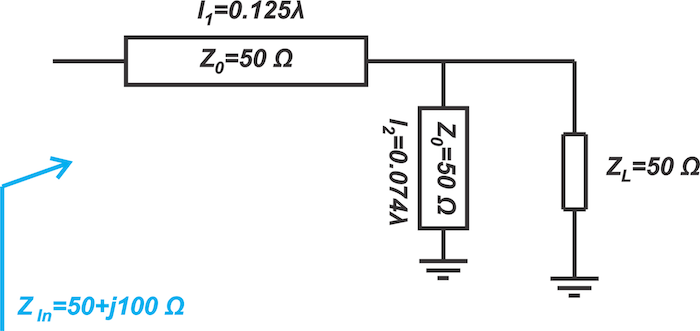##### Figure 7. Example diagram where the stub is placed at the load end of the line.

Again both l1 and l2 need to be adjusted to account for variations in ZL. It is inconvenient to adjust l1 because it is placed between the load and input ports. However, adjusting the length of the stub l2 can be relatively easier, particularly when using short-circuited stubs. This type of adjustable stub can be created by means of a sliding short that moves along a coaxial line. This observation is the basis of a matching circuit known as the double stub tuner, which is shown in Figure 8.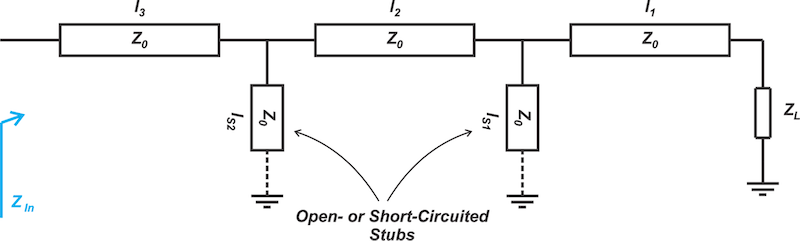##### Figure 8. Diagram of a matching circuit or double stub tuner.

In this case, the length of the series sections between the input and output ports is fixed (l1, l2, and l3 are constant), but the stub lengths ls1 and ls2 are adjustable. Note that by fixing the length of the series line, we are losing one degree of freedom in our design. To compensate for this, two adjustable stubs, rather than a single stub, are used. This gives us two degrees of freedom, similar to the case of a single stub tuner. Therefore, while the position of both stubs is fixed, their length is adjustable. From here, the stubs can be terminated in an open or short circuit.

Before explaining the workings of a double stub tuner, it is helpful to examine how a series line can rotate a given impedance contour on the Smith chart.

### Rotating an Impedance Contour Through a Series Line

We know that moving away from a termination on a transmission line produces a clockwise rotation along the load’s constant |Γ| circle. If the line’s electrical length is θ, we’ll have a rotation of 2θ on the Smith chart (Figure 9).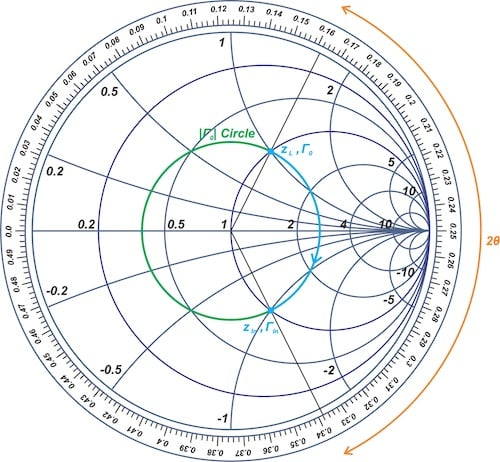##### Figure 9. Example Smith chart with a rotation of 2θ.

Rather than rotating a single point, the above transformation can also evidently rotate a given contour on the Smith chart. For example, assume that the locus of a load admittance yL is the constant-conductance circle of g = 1 (the blue circle in Figure 10).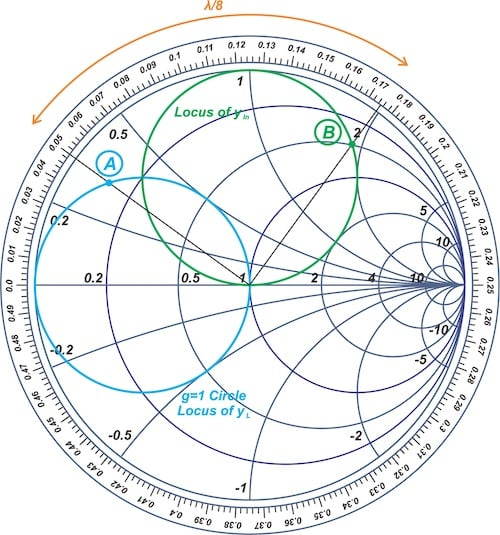##### Figure 10. Smith chart showing the locus of a load admittance yL.

If we terminate a λ/8 line in yL, the locus of the admittance seen at the input of the line can be obtained by rotating the g = 1 circle in a clockwise direction by λ/8 (or 2 × 45° = 90°), leading to the green circle in the above figure.

As can be seen, each arbitrary point on the blue circle is rotated by λ/8 to produce its corresponding point on the green circle (e.g. point A is transformed to point B). Therefore, a series line rotates the whole contour.

### How Does a Double Stub Tuner Work?

Consider the double stub matching network shown in Figure 11.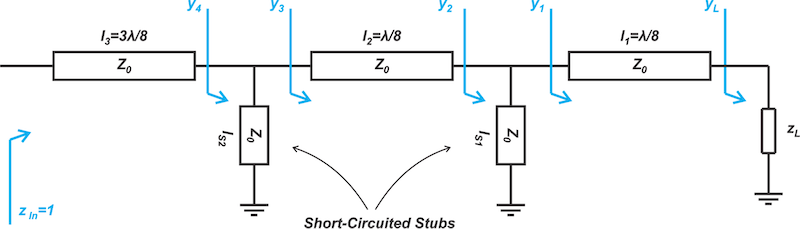##### Figure 11. Example double stub matching network.

In this case, we have l1 = l2 = λ/8, l3 = 3λ/8, and the stubs are terminated in short circuits. The goal is to transform an arbitrary load impedance, zL, to a normalized impedance of zin = 1. The admittance seen at the input port is yin = 1. Since yin is at the center of the Smith chart, so is y4. If the susceptance of the stub of length ls2 is jbs2, we have y= 1 - jbs2. In other words, y3 is on the g = 1 constant-conductance circle.

From our previous discussion, we know that the middle series line, which is of length l2, rotates y2 in a clockwise direction by twice the electrical length of the line to produce y3. Therefore, we just need to rotate y3 in a counterclockwise direction by λ/8 to find the locus of y2. The contours of y3 and y2 are shown by the green and blue circles in Figure 12.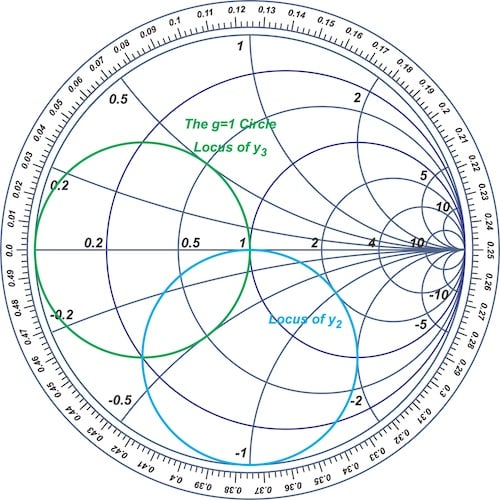##### Figure 12. Smith chart showing the contours of y3 and y2.

The above explanation shows that if y2 is on the blue circle, y3 will be on the green circle because l2 is equal to λ/8. In this case, a properly selected stub length ls2 can move y4 to the center of the Smith chart. Therefore, we choose the length of the first stub ls1 to move y2 to somewhere on the blue circle and choose ls1 to bring y4 to the center of the Smith chart.

Note that the position of the blue circle on the Smith chart is determined by the length of the middle series line, l2. This circle is often referred to as the “rotated 1 + jb circle” or “rotated g = 1 circle” in the discussion of double stub tuning. Let’s look at an example.

### Example 2: Design a Double Stub Matching Network

Design the double stub matching network of Figure 11 to transform Z= 100 + j50 Ω to ZIn = 50 Ω. As shown in the circuit diagram, we have l= l= λ/8 and l= 3λ/8, and the stubs are terminated in short circuits. If the load impedance is a series RL circuit and the match frequency is 1 GHz, plot the reflection coefficient magnitude versus frequency.

The normalized load admittance, yL,—corresponding to z= 2 + j—is marked on the Smith chart of Figure 13.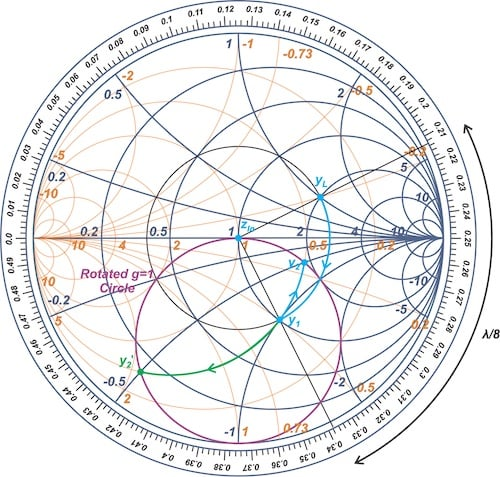A clockwise rotation of λ/8 due to the series line on the load side transforms yL to y1, as depicted above.

In this example, y1 is on the g = 0.5 circle, and the susceptance added by the first stub should move us to the intersection of the g = 0.5 circle with the rotated g = 1 circle (the purple circle). With that in mind, there are two possible solutions, denoted by y2 and y2′. In this example, we'll choose to use the path through y2. Next, the middle series line produces a λ/8 clockwise rotation and brings us to y3 on the g = 1 circle (Figure 14).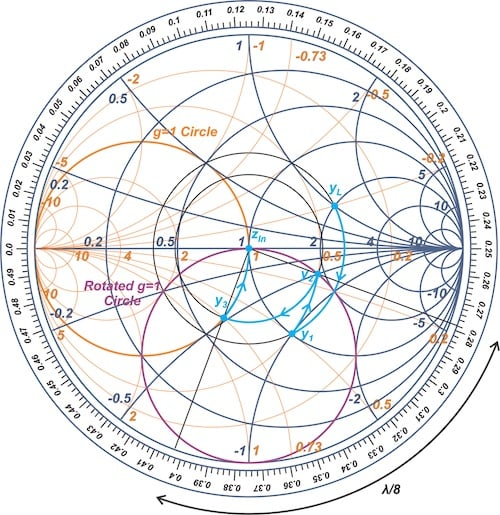##### Figure 14. Smith chart showing how the middle series line produces a λ/8 clockwise rotation and brings us to y3 on the g = 1 circle.

Finally, the second stub adds the appropriate value of susceptance jbs2 to move us to the center of the Smith chart. Figure 15 shows the final path with the required constant-susceptance arcs so we can calculate ls1 and ls2.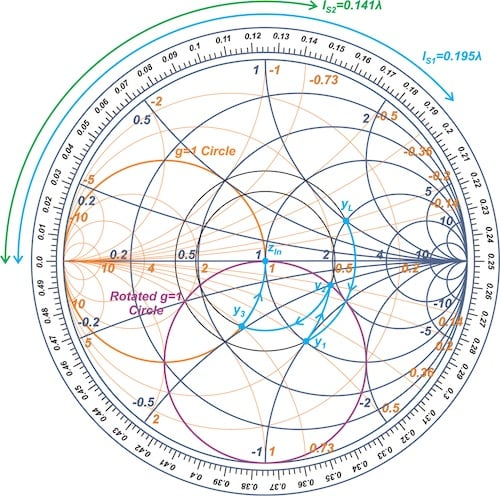##### Figure 15. Smith chart showing the final path with the required constant-susceptance arcs.

The susceptance of the first stub is equal to the susceptance difference between y2 and y1, leading to bs1 = j0.14 - j0.5 = -j0.36. As shown in the figure, a short-circuited stub with a length of ls1 = 0.195λ produces the susceptance bs1 = -j0.36. Also, the second stub should produce a susceptance equal to bs2 = -j0.73 to move us to the center of the Smith chart. This requires a short-circuited stub of length ls2 = 0.141λ, as shown in Figure 15. This completes the design.

Since the Z= 100 + j50 Ω is said to be a series RL circuit and the match frequency is 1 GHz, we obtain R = 100 Ω and L = 7.96 nH. The input reflection coefficient (S11) of the circuit is shown in Figure 16, which confirms a good match at 1 GHz.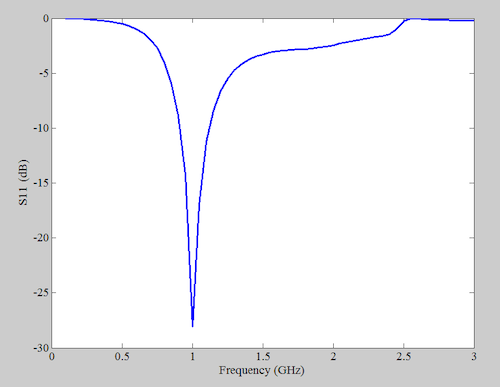### The Smith Chart Forbidden Region

The above design process is based on the assumption that the admittance added by the first stub can move y2 to a point on the rotated g = 1 circle. This might not be possible for certain values of yL and l1. In the above example, if y1 falls inside the g = 2 circle, adding a susceptance cannot transform it to a point on the rotated g = 1 circle. This is illustrated below in Figure 17.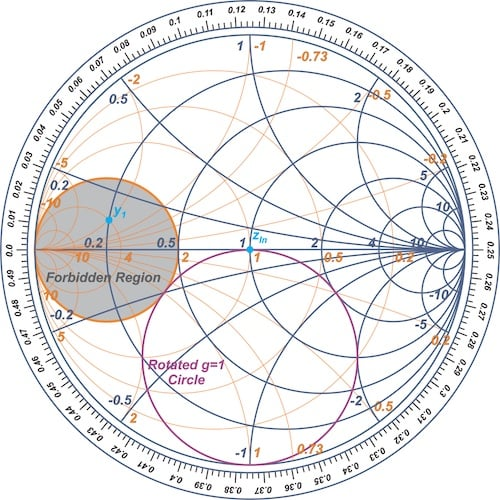##### Figure 17. Smith chart showing the "forbidden region."

That’s why the region inside the g = 2 circle is a forbidden region in our example. To get around this, commercial double stub tuners choose l= l± λ/4, which you can learn more about in this recommended book "RF Circuit Design: Theory and Applications" by Reinhold Ludwig and Gene Bogdanov. In this case, if a load falls inside the forbidden region, one can simply connect the load to the opposite end of the tuner to move y1 out of the forbidden region.

### Designing Through a Z Smith Chart

Rather than using a ZY Smith chart, it is possible to perform the above calculations using only an impedance Smith chart (or a Z Smith chart). In this case, the Z Smith chart is interpreted as a Y Smith chart. You can find an example of this in David M. Pozar’s well-known book “Microwave Engineering.”

As a final note, it is worthwhile to mention that tuning a double stub tuner is usually done by trial and error in practice—watch this video for an example. This is usually faster than computing and then implementing an analytical solution.